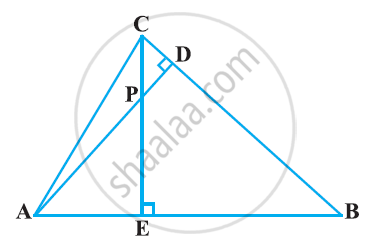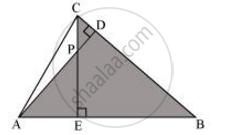# In the following figure, altitudes AD and CE of ΔABC intersect each other at the point P. Show that - Mathematics

In the following figure, altitudes AD and CE of ΔABC intersect each other at the point P. Show that:ΔABD ∼ ΔCBE

#### SolutionIn ΔABD and ΔCBE,

∠ABD = ∠CBE (Common)

Hence, by using AA similarity criterion,

ΔABD ∼ ΔCBE

Concept: Criteria for Similarity of Triangles
Is there an error in this question or solution?

#### APPEARS IN

NCERT Class 10 Maths
Chapter 6 Triangles
Exercise 6.3 | Q 7.2 | Page 140